# Geometry ConstructionsRebecca Baker
question

Perpendicular bisector construction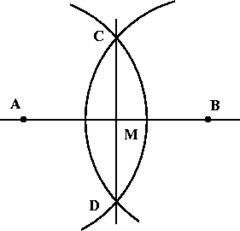question

Perpendicular line through a point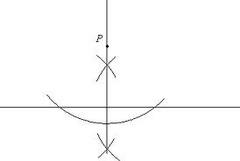question

Copy an angle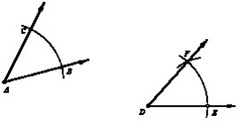question

Construct an angle bisector.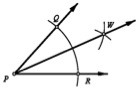question

Parallel line through a point constructionmake a perpendicular line through the point then make a perpendicular line through the new line. Use patty paper
question

Copying a Segment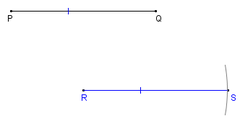question

median construction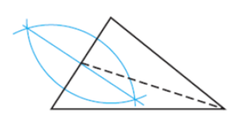Find the midpoint of one side then connect the opposite vertex
question

Altitude Construction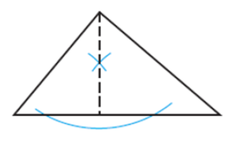Make the perpendicular from the vertex to the opposite side.
question

midsegment construction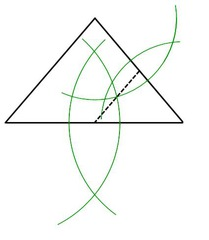find the median of two sides then connect the midpoints together.
question

constructing the midpoint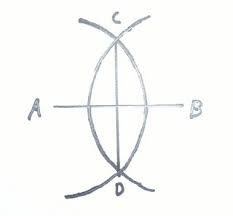construct the perpendicular, instead of drawing the line
question

orthocenter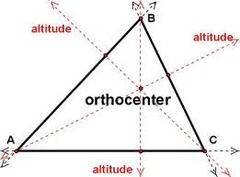construction of the 3 altitudes
question

Circumcenter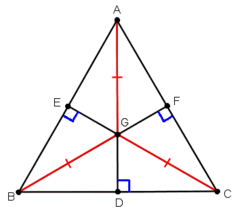Construction of the 3 perpendicular bisectors
question

incenter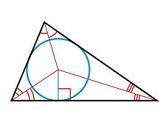construction of the 3 angle bisectors
question

centroid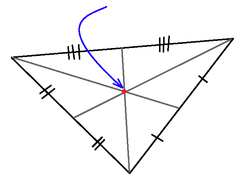constructions of the three medians
question

circumscribed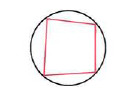circle outside the shape
question

inscribed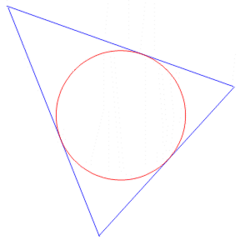circle inside the shape
question

concurrency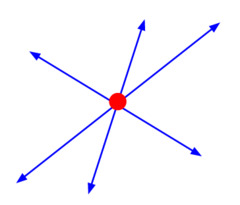point where the 3 or more lines meet
question

median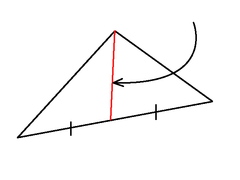A line segment connecting a vertex of a triangle to the midpoint of the opposite side
question

Altitude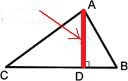A perpendicular segment from a vertex to the opposite side
question

Perpendicular Bisector